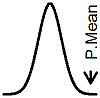P.Mean: Calculating a confidence interval for a standard deviation (created 2011-07-10). News: Sign up for "The Monthly Mean," the newsletter that dares to call itself average, www.pmean.com/news.Suppose that you had a sample of 80 observations and you computed a standard deviation of those 80 observations. Like any other statistic, the standard deviation will have some sampling error associated with it. But how much sampling error. This is an important question, because I needed to establish that 80 was a reasonable sample size in a study where there was no formal research hypothesis. You do this by showing that key statistics that you will estimate have good precision. In this study, the standard deviation was especially important, so I wanted to show that a standard deviation based on 80 observations had a good level of precision.

It's a standard result in mathematical statistics that the sample variance (variance is the square of the standard deviation) is distributed as a multiple of a Chi-square random variable. To be more precise, define the greek letter sigma (σ) as the unknown standard deviation of the population and the roman letter S as the standard deviation computed from a sample of n observations.

If the data comes from a normally distributed population, then n-1 times the sample variance divided by the population variance has a Chisquare distribution with n-1 degrees of freedom.Now if the data comes from a non-normally distributed population, you still may be okay. If the sample size is large and if certain other conditions exist, then the above distribution will hold approximately.

Now if the ratio follows a Chi-square distribution, then you can make the following probability statement.Re-arrange the terms algebraicly to getThe two limitsandrepresent a 95% confidence interval for the sample variance. Take the square root of both sidesandto get a 95% confidence interval for the sample standard deviation.

There's a subtle shift that happened in our algebraic manipulations that appears in the final formula. Notice that the upper percentile (the 1-alpha/2 percentile) of the Chi-square distribution appears in the denominator of the lower confidence limit. This happened because in an intermediate step of the algebraic manipulation not shown here, you need to invert the fraction and at the same time invert the inequality (if A < B < C then 1/C < 1/B < 1/A). It make sense, of course, because the numerators are the same. So lower limit has to have a larger number in the denominator than the upper limit.

Something else interesting is going on. Most confidence intervals that you have seen are additive--your interval is that sample estimate plus or minus a certain value. This confidence interval, though, is multiplicative--your interval is the sample estimate multiplied by a couple of values.

In our example, there were 80 observations. The appropriate limits of the Chi-square distribution areandThe upper and lower limits of the 95% confidence interval for the standard deviation, then areandNotice that I put the larger Chisquare percentile in the denominator of the lower confidence limit. Our confidence interval is 86% to 118% of the sample standard deviation, which indicates a small amount of sampling error.This page was written by Steve Simon and is licensed under the Creative Commons Attribution 3.0 United States License. Need more information? I have a page with general help resources. You can also browse for pages similar to this one at Confidence Intervals.